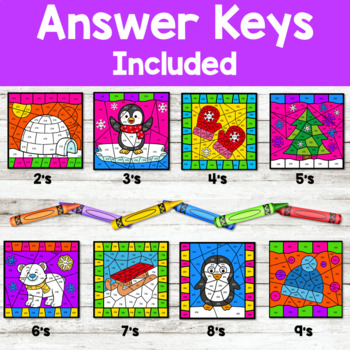# Division Color by Code | {2’s - 9's} {Winter}Subject
Resource Type
File Type

PDF

(28 MB|23 pages)
Product Rating
3.8
(7 Ratings)
Standards
• Product Description
• StandardsNEW

Your students will love these color by code worksheets while getting the essential math fact fluency practice they need.

The color by code pages are easy prep – just print and go! Each page focuses on a different division table, which makes differentiation a snap!

Perfect for:

• Independent Practice Seatwork

• Math Centers

• Review

• Homework

• Morning Work

• RTI pull out

INCLUDED RESOURCES ★

• 8 Color-by-Code (B&W)

• 8 Color-by-Code answer pages (Color)

Customer Tips:

♥ Click on the ★ above (by my picture) to follow my store.

♥ Leave feedback to earn credit pointsto save money on future products!

------------------------------------------------------------------

Let’s Connect:

Pinterest

Instagram

Peggy @ Primary Flourish

------------------------------------------------------------------

Fluently multiply and divide within 100, using strategies such as the relationship between multiplication and division (e.g., knowing that 8 × 5 = 40, one knows 40 ÷ 5 = 8) or properties of operations. By the end of Grade 3, know from memory all products of two one-digit numbers.
Apply properties of operations as strategies to multiply and divide. Examples: If 6 × 4 = 24 is known, then 4 × 6 = 24 is also known. (Commutative property of multiplication.) 3 × 5 × 2 can be found by 3 × 5 = 15, then 15 × 2 = 30, or by 5 × 2 = 10, then 3 × 10 = 30. (Associative property of multiplication.) Knowing that 8 × 5 = 40 and 8 × 2 = 16, one can find 8 × 7 as 8 × (5 + 2) = (8 × 5) + (8 × 2) = 40 + 16 = 56. (Distributive property.)
Determine the unknown whole number in a multiplication or division equation relating three whole numbers. For example, determine the unknown number that makes the equation true in each of the equations 8 × ? = 48, 5 = __ ÷ 3, 6 × 6 = ?.
Use multiplication and division within 100 to solve word problems in situations involving equal groups, arrays, and measurement quantities, e.g., by using drawings and equations with a symbol for the unknown number to represent the problem.
Interpret products of whole numbers, e.g., interpret 5 × 7 as the total number of objects in 5 groups of 7 objects each. For example, describe a context in which a total number of objects can be expressed as 5 × 7.
Total Pages
23 pages
Included
Teaching Duration
N/A
Report this Resource to TpT
Reported resources will be reviewed by our team. Report this resource to let us know if this resource violates TpT’s content guidelines.